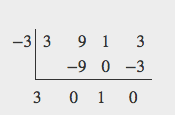## Use the Fundamental Theorem of Algebra

Now that we can find rational zeros for a polynomial function, we will look at a theorem that discusses the number of complex zeros of a polynomial function. The Fundamental Theorem of Algebra tells us that every polynomial function has at least one complex zero. This theorem forms the foundation for solving polynomial equations.

Suppose f is a polynomial function of degree four, and $f\left(x\right)=0$. The Fundamental Theorem of Algebra states that there is at least one complex solution, call it ${c}_{1}$. By the Factor Theorem, we can write $f\left(x\right)$ as a product of $x-{c}_{\text{1}}$ and a polynomial quotient. Since $x-{c}_{\text{1}}$ is linear, the polynomial quotient will be of degree three. Now we apply the Fundamental Theorem of Algebra to the third-degree polynomial quotient. It will have at least one complex zero, call it ${c}_{\text{2}}$. So we can write the polynomial quotient as a product of $x-{c}_{\text{2}}$ and a new polynomial quotient of degree two. Continue to apply the Fundamental Theorem of Algebra until all of the zeros are found. There will be four of them and each one will yield a factor of $f\left(x\right)$.

### A General Note: The Fundamental Theorem of Algebra states that, if f(x) is a polynomial of degree n > 0, then f(x) has at least one complex zero.

We can use this theorem to argue that, if $f\left(x\right)$ is a polynomial of degree $n>0$, and a is a non-zero real number, then $f\left(x\right)$ has exactly n linear factors

$f\left(x\right)=a\left(x-{c}_{1}\right)\left(x-{c}_{2}\right)…\left(x-{c}_{n}\right)$

where ${c}_{1},{c}_{2},…,{c}_{n}$ are complex numbers. Therefore, $f\left(x\right)$ has n roots if we allow for multiplicities.

### Q & A

Does every polynomial have at least one imaginary zero?

No. A complex number is not necessarily imaginary. Real numbers are also complex numbers.

### Example 6: Finding the Zeros of a Polynomial Function with Complex Zeros

Find the zeros of $f\left(x\right)=3{x}^{3}+9{x}^{2}+x+3$.

### Solution

The Rational Zero Theorem tells us that if $\frac{p}{q}$ is a zero of $f\left(x\right)$, then p is a factor of 3 and q is a factor of 3.

$\begin{cases}\frac{p}{q}=\frac{\text{factor of constant term}}{\text{factor of leading coefficient}}\hfill \\ \text{ }=\frac{\text{factor of 3}}{\text{factor of 3}}\hfill \end{cases}$

The factors of 3 are $\pm 1$ and $\pm 3$. The possible values for $\frac{p}{q}$, and therefore the possible rational zeros for the function, are $\pm 3,\text{\pm 1, and }\pm \frac{1}{3}$. We will use synthetic division to evaluate each possible zero until we find one that gives a remainder of 0. Let’s begin with –3.Dividing by $\left(x+3\right)$ gives a remainder of 0, so –3 is a zero of the function. The polynomial can be written as

$\left(x+3\right)\left(3{x}^{2}+1\right)$

We can then set the quadratic equal to 0 and solve to find the other zeros of the function.

$\begin{cases}3{x}^{2}+1=0\hfill \\ \text{ }{x}^{2}=-\frac{1}{3}\hfill \\ \text{ }x=\pm \sqrt{-\frac{1}{3}}=\pm \frac{i\sqrt{3}}{3}\hfill \end{cases}$

The zeros of $f\left(x\right)$ are –3 and $\pm \frac{i\sqrt{3}}{3}$.

### Analysis of the Solution

Look at the graph of the function f. Notice that, at $x=-3$, the graph crosses the x-axis, indicating an odd multiplicity (1) for the zero $x=-3$. Also note the presence of the two turning points. This means that, since there is a 3rd degree polynomial, we are looking at the maximum number of turning points. So, the end behavior of increasing without bound to the right and decreasing without bound to the left will continue. Thus, all the x-intercepts for the function are shown. So either the multiplicity of $x=-3$ is 1 and there are two complex solutions, which is what we found, or the multiplicity at $x=-3$ is three. Either way, our result is correct.Figure 2

### Try It 4

Find the zeros of $f\left(x\right)=2{x}^{3}+5{x}^{2}-11x+4$.

Solution